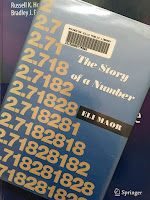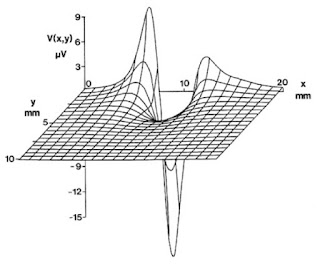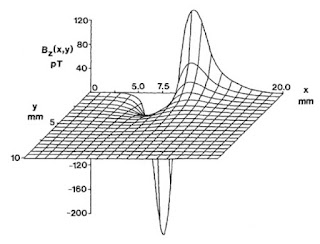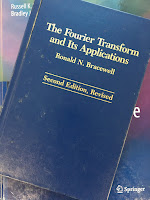Friday, May 27, 2011

e, The Story of a Number

On page 33 of the 4th edition of Intermediate Physics for Medicine and Biology, Russ Hobbie and I introduce the constant e.
The number e is approximately equal to 2.71828… and is called the “base of the natural logarithms.” Like π (3.14159…) e has a long history [Maor (1994)].e: The Story of a Number, by Eli Maor.
The citation is to the delightful book e: The Story of a Number, by Eli Maor. In his preface, Maor explains why he wrote the book.
My goal is to tell the story of e on a level accessible to readers with only a modest background in mathematics. I have minimized the use of mathematics in the text itself, delegating several proofs and derivations to the appendixes. Also, I have allowed myself to digress from the main subject on occasion to explore some side issues of historical interest. These include biographical sketches of the many figures who played a role in the history of e, some of whom are rarely mentioned in textbooks. Above all, I want to show the great variety of phenomena—from physics and biology to art and music—that are related to the exponential function ex, making it a subject of interest in fields well beyond mathematics.
Our Chapter 2, about exponential growth, centers on the exponential and logarithm functions, and our Appendix C lists many of the properties of these functions. Maor explores all sorts of interesting facts about e. For instance, 878/323 is a very good rational approximation to this irrational number. You can recall the first ten digits of e by remembering 2.7 (Andrew Jackson)2 [Jackson was elected president in 1828]. In his Chapter 13, Maor presents some beautiful continued fractions for e that I will not attempt to reproduce here using html.

When developing the Fourier series in Chapter 11 of Intermediate Physics for Medicine and Biology, Russ and I note that “the remarkable property of imaginary numbers that make them useful in this context is that e = cosθ + i sinθ.” (Here, i is the square root of minus one.) Maor sets θ = π to obtain an equation studied by the Swiss mathematician Leonhard Euler

e = −1 ,

and claims
it must surely rank among the most beautiful formulas in all of mathematics. Indeed, by rewriting it as eπi + 1 = 0, we obtain a formula that connects the five most important constants of mathematics (and also the three most important mathematical operation—addition, multiplication, and exponentiation). These five constants symbolize the four major branches of classical mathematics: arithmetic, represented by 0 and 1; algebra, by i; geometry, by π; and analysis, by e.
Taking a less aesthetic view, Russ and I downplay the use of complex exponentials in Intermediate Physics for Medicine and Biology.
The Fourier transform is usually written in terms of complex exponentials. We have avoided using complex exponentials. They are not necessary for anything done in this book. The sole advantage of complex exponentials is to simplify the notation. The actual calculations must be done with real numbers.
Another reason I often steer clear of complex exponentials is that I place great importance on being able to visualize physically what a mathematical expression is saying, and I find trigonometric functions far easier to envision than complex exponentials. So, while I concede the abstract beauty of the formula e = −1, I don’t find it so useful when thinking about physics.

While educating his readers about e, Maor also introduces them to many famous mathematicians, including Archimedes, Napier, Newton, Gauss, the Bernoullis, and above all Euler, who is apparently one of Maor’s favorites.
Leonhard Euler (1707–1783) is unquestionably the Mozart of mathematics, a man whose immense output--not yet published in full—is estimated to fill at least seventy volumes. Euler left hardly an area of mathematics untouched, putting his mark on such diverse fields as analysis, number theory, mechanics and hydrodynamics, cartography, topology, and the theory of lunar motion.
Maor discusses the uses of logarithms and exponentials in biology. He talks about the logarithmic spiral and its role in growth, for instance, of a nautilus shell. He also makes an interesting comparison between the ear and the eye.
The remarkable sensitivity of the human ear to frequency changes is matched by its audibile range—from about 20 cycles per second to about 20,000 (the exact limits vary somewhat with age). In terms of pitch, this corresponds to about ten octaves (an orchestra rarely uses more then seven). By comparison, the eye is sensitive to a wavelength range from 4,000 to 7,000 angstroms (10−8 cm)—a range of less than two “octaves.” [Doesn’t Maor mean: less than one “octave”?]
I’m particularly fond of Maor’s recreation of a meeting between Bach and one of the Bernoulli’s
Let us imagine a meeting between Johann Bernoulli (Johann I, that is) and Johann Sebastian Bach. The year is 1740. Each is at the peak of his fame. Bach, at the age of fifty-five, is organist, composer, and Kapellmeister (musical director) at St. Thomas’s Church in Leipzig. Bernoulli, at seventy three, is the most distinguished professor of the University of Basel.
The resulting imagined conversation is fascinating and amusing. Musicians interested in the “equal tempered scale” will enjoy this section.

I will close this blog entry the same way Maor ended his book, by letting e take a final bow. Here is e to one hundred decimal places:

2.7182818284590452353
60287471352662497757
24709369995957496696
76277240766303535475
94571382178525166427

Friday, May 20, 2011

Non-Newtonian Fluids and the Rheology of Blood

In Chapter 1 of the 4th edition of Intermediate Physics for Medicine and Biology, Russ Hobbie and I explain the difference between a Newtonian fluid and a non-Newtonian fluid.
A fluid can support a viscous shear stress if the shear strain is changing. One way to create such a situation is to immerse two parallel plates, each of area S, in the fluid, and to move one parallel to the other … The variation of velocity between the plates gives rise to a velocity gradient dvx/dy

In order to keep the top plate moving and the bottom plate stationary, it is necessary to exert a force of magnitude F on each plate: to the right on the upper plate and to the left on the lower plate. The resulting shear stress or force per unit area is in many cases proportional to the velocity gradient:

F/S = η dvx/dy .   (1.33)

The constant η is called the coefficient of viscosity … Fluids that are described by Eq. 1.33 are called Newtonian fluids. Many fluids are not Newtonian.
At the end of the chapter, we give an example of a biologically important non-Newtonian fluid.
Blood is not a Newtonian fluid. The viscosity depends strongly on the fraction of volume occupied by red cells (the hematocrit).
An excellent review of blood’s fluid behavior can be found in the article “Rheology of Blood” by Edward Merrill (Physiological Reviews, Volume 49, Pages 863–888, 1969). Rheology is the part of fluid mechanics that deals with non-Newtonian fluids. Merrill explains clearly the difference between a Newtonian fluid with a high viscosity and a Non-Newtonian fluid.
A Newtonian liquid is one in which the viscosity, at fixed temperature and pressure, is independent of the shear stress. Thus, a non-Newtonian liquid is one in which the viscosity depends on shear stress. Water and honey are Newtonian, but many aqueous suspensions of fine particulate matter such as water-base paint, plaster, and oil emulsions are non-Newtonian. The distinction is qualitatively obvious if one imagines two spoons, one in a pot of honey (Newtonian) and the other in a pot of mayonnaise (non-Newtonian emulsion). The honey is harder to stir (has a higher viscosity) than the mayonnaise, but when the spoons are removed and held above the pots, the honey continues to drizzle off its spoon, whereas the mayonnaise coating the other spoon clings indefinitely to it without flow, thus exhibiting “infinite” viscosity.
An important concept when discussing the rheology of blood is yield stress. Merrill explains
Blood … exhibits a “yield stress.” This means that, if …one increases from zero the stress, but keeps it less than a critical value, the response will be elastic … On removal of the stress, the shape of the blood film will be unaltered, i.e., no flow will have occurred. However, if the yield stress is exceeded, irreversible deformation will occur.
In other words, it acts like a solid at low stress, and a fluid at high stress. Merrill concludes by discussing the physiological significance of the non-Newtonian nature of blood.
In summary, the relevance of blood rheology to physiological fluid mechanics is to make stopping of flows easier, starting of flows more difficult, and slow flows more energy consuming than would be expected if blood were a simple, cell-less, micromolecular fluid of equal viscosity—and these effects are increasingly emphasized with increase of hematocrit and fibrinogen concentration.
Besides blood, another dramatic example of a non-Newtonian fluid is a mixture of corn starch and water. My Oakland University colleague Alberto Rojo (whose office is next door to mine) has made a fun video demonstrating how you can “walk on water” by taking advantage of this mixture’s non-Newtonian properties. The effect is fascinating.

Alberto Rojo walks on a mixture of corn starch and water.

Friday, May 13, 2011

Drawing Figures

Two of my favorite figures in the 4th edition of Intermediate Physics for Medicine and Biology are Fig. 7.13 (the extracellular potential produced by an action potential along a nerve axon) and Fig. 8.14 (the magnetic field produced by the same axon). John Wikswo and I prepared these figures when I was in graduate school at Vanderbilt University.Fig. 7.13. The exterior potential calculated using the method of Clark and Plonsey. From Intermediate Physics for Medicine and Biology, 4th edition.Fig. 8.14. A three-dimensional plot of the magnetic field around the crayfish axon. From Intermediate Physics for Medicine and Biology, 4th edition.

The figures appeared in the second edition of Russ’s book, and have continued on through subsequent editions (including the 4th edition, on which I have the high honor of becoming a coauthor). Figures like that required much time and expense to prepare, and are difficult to edit. But my, it was more fun to really “draw” those figures than it is to churn out figures using graphics software.

Friday, May 6, 2011

Central Slice Theorem and Ronald Bracewell

Chapter 12 of the 4th edition of Intermediate Physics for Medicine and Biology deals with images and tomography. One of the key ideas in tomography is the “central slice theorem.” Russ Hobbie and I write in Section 12.4 that
The Fourier transform of the projection at angle θ is equal to the two-dimensional Fourier transform of the object, evaluated in the direction θ in Fourier transform space. This result is known as the projection theorem or the central slice theorem (Problem 17). The transforms of a set of projections at many different angles provide values of C and S [the cosine and sine parts of the 2-d Fourier transform] throughout the kxky plane [frequency space] that can be used in Eq. 12.9a [the definition of the 2-d Fourier transform] to calculate f(x,y).
I consider the central slice theorem to be one of the most important concepts in medical imaging. How was this fundamental idea first developed? The answer to that question provides a fascinating example of how physics and engineering can contribute to medicine.

Ronald Bracewell first developed the central slice theorem while working in the field of radio astronomy. His 2007 New York Times obituary states
Ronald N. Bracewell, an astronomer and engineer who used radio telescopes to make early images of the Sun’s surface, in work that also led to advances in medical imaging, died on Aug. 12 at his home in Stanford, Calif. He was 86…

With his colleagues at Stanford University in the 1950s, Dr. Bracewell designed a specialized radio telescope, called a spectroheliograph, to receive and evaluate microwaves emitted by the Sun…

Later, in the 1970s, the techniques and a formula devised by Dr. Bracewell were applied by other scientists in developing X-ray imaging of tumors, called tomography, and other forms of medical imaging that scan electromagnetic and radio waves. Dr. Bracewell advised researchers at Stanford and other institutions, but did not conduct laboratory research in the field.The Fourier Transform and Its Applications, by Ronald Bracewell.
Russ and I cite Bracewell’s 1990 paper “Numerical Transforms” (Science, Volume 248, Pages 697–704). The central slice theorem was published in 1956 in the Australian Journal of Physics (Volume 9, Pages 198–217). Early in this career Bracewell published a lot in that journal, which is now defunct but maintains a website with free access to all the papers. Bracewell also wrote a marvelous book: The Fourier Transform and Its Applications (originally published in 1965, the revised 2nd edition is published by McGraw-Hill, New York, 1986). When writing this blog entry, I checked this book out of Kresge Library here at Oakland University. Once I opened it, I realized it is an old friend. I am sure I read this book in graduate school. It contains many pictures that allow the student to gain an intuition about the Fourier transform; an extraordinarily valuable skill to develop. The introduction states
The present work began as a pictorial guide to Fourier transforms to complement the standard lists of pairs of transforms expressed mathematically. It quickly became apparent that the commentary would far outweigh the pictorial list in value, but the pictorial dictionary of transforms is nevertheless important, for a study of the entries reinforces the intuition, and many valuable and common types of function are included which, because of their awkwardness when expressed algebraically, do not occur in other lists.
The text also does a fine job describing convolutions.
Convolution is used a lot here. Experience shows that it is a fairly tricky concept when it is presented bluntly under its integral definition, but it becomes easy if the concept of a functional is first understood.
Many of the ideas that Russ and I present in Chapter 11 of Intermediate Physics for Medicine and Biology are examined in more detail in Bracewell’s book. I recommend it as a reference to keep at your side as your plow through the mathematics of Fourier analysis.

Finally, Bracewell’s view of homework problems, as stated in his Preface to the second edition, mirrors my own.
A good problem assigned at the right stage can be extremely valuable for the student, but a good problem is hard to compose. Among the collection of supplementary problems now included at the end of the book are several that go beyond being mathematical exercises by inclusion of technical background or by asking for opinions.